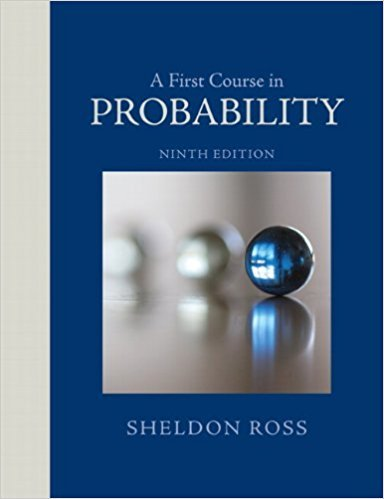×
Get Full Access to A First Course In Probability - 9 Edition - Chapter 2 - Problem 34p
Get Full Access to A First Course In Probability - 9 Edition - Chapter 2 - Problem 34p

×

# The second Earl of Yarborough is reported to have bet atISBN: 9780321794772 63

## Solution for problem 34P Chapter 2

A First Course in Probability | 9th Edition

• Textbook Solutions
• 2901 Step-by-step solutions solved by professors and subject experts
• Get 24/7 help from StudySoup virtual teaching assistantsA First Course in Probability | 9th Edition

4 5 1 367 Reviews
31
2
Problem 34P

Problem 34P

The second Earl of Yarborough is reported to have bet at odds of 1000 to 1 that a bridge hand of 13 cards would contain at least one card that is ten or higher. (By ten or higher we mean that a card is either a ten, a jack, a queen, a king, or an ace.) Nowadays, we call a hand that has no cards higher than 9 a Yarborough. What is the probability that a randomly selected bridge hand is a Yarborough?

Step-by-Step Solution:

Solution:

Step 1 of 2:

It is given that bridge hand of 13 cards contains at least one card that is ten or higher.

Also it is given that Yarborough is the hand that has no cards higher than 9.

Using this we need to find the probability that a randomly selected bridge hand is a Yarborough.

Step 2 of 2

##### ISBN: 9780321794772

Unlock Textbook Solution